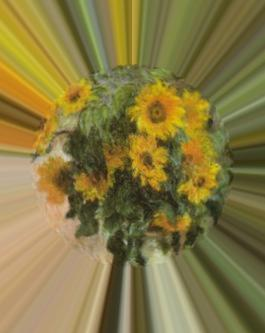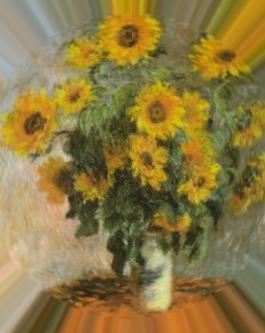## STRIATIONS

 Applies radial or circular striations to image.

 USAGE: striations [-r radius ] [-c center] infile outfile USAGE: striations [-h or -help] -t .... type ............. type of striations; choices are radial (or r) .......................... or circular (c); default=radial -r .... radius ........... radius from center point where striations begin; .......................... integer>=0; default is half the minimum image dimension -c .... center ........... center point for striation effect; center=cx,cy; .......................... integer>=0; default is center of image -k .... column ........... column to use for circular striations; integers; .......................... 0<=column=0. The default is half the minimum dimension of the image. -c center ... CENTER=cx,cy are the comma separated coordinates in the image from where the radial striations eminate. Values are integers>=0. The default is the center of the image. -k column ... COLUMN of the polar image to use for generating the circular striations. Values are integers, such that 0<=column

### EXAMPLES

 NOTE: The radial formula has been corrected. But I have not modified the examples. So the radius of the effect may be slightly different if one repeats the examples.

 Radial Striations OriginalArguments: -t r -r 50 Arguments: -t r -r 100Arguments: -t r -r 150 Arguments: -t r -r 200Circular Striations OriginalArguments: -t c -r 50 Arguments: -t c -r 100Arguments: -t c -r 100 -k 130 Arguments: -t r -r 150What the script does is as follows: Converts image to polar coordinates Crops the height as determined by the radius Repeats the last cropped row to fill the height back to original size Converts back to rectangular coordinates This is equivalent to the following IM commands ww=`convert \$infile -format "%[fx:w]" info:` hh=`convert \$infile -format "%[fx:h]" info:` rad1=`convert \$infile -format "%[fx:floor(2*\$rad*h/sqrt(w*w+h*h))]" info:` hmr=`convert xc: -format "%[fx:max(1,\$hh-\$rad1)]" info:` convert \$infile -distort depolar -1,0,\$cx,\$cy \$infile convert \$tmpA1[\${ww}x\${rad1}+0+0] \ \( \$tmpA1[\${ww}x1+0+\${rad1}] -scale \${ww}x\${hmr}! \) \ -append -crop \${ww}x\${hh}+0+0 +repage \ -distort polar -1,0,\$cx,\$cy \$outfile